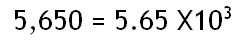Home Math Calculators Math  Flashcards Math Games Math  Homework Math Lessons Math Terms Math Worksheets Math Tools
You Are In Math Glossary of Terms

# Scientific Notation

A way of writing a very large or very small numbers using a number between 1 and 10 multiplied by a power of ten.

Example:If you know that there are 496 students in your school, you can say that there are approximately 500 students in your school or 5.68, after rounding will be 6.